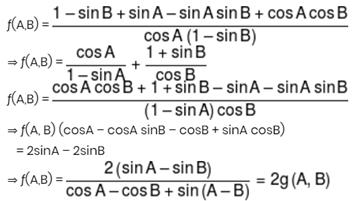Courses

# JEE Main Mathematics Mock - 1

## 25 Questions MCQ Test Mock Test Series for JEE Main & Advanced 2022 | JEE Main Mathematics Mock - 1

Description
This mock test of JEE Main Mathematics Mock - 1 for JEE helps you for every JEE entrance exam. This contains 25 Multiple Choice Questions for JEE JEE Main Mathematics Mock - 1 (mcq) to study with solutions a complete question bank. The solved questions answers in this JEE Main Mathematics Mock - 1 quiz give you a good mix of easy questions and tough questions. JEE students definitely take this JEE Main Mathematics Mock - 1 exercise for a better result in the exam. You can find other JEE Main Mathematics Mock - 1 extra questions, long questions & short questions for JEE on EduRev as well by searching above.
QUESTION: 1

Solution:
QUESTION: 2

Solution:
QUESTION: 3

### If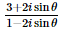sin θ is real, then θ =

Solution:
QUESTION: 4

In the following question, a Statement of Assertion (A) is given followed by a corresponding Reason (R) just below it. Read the Statements carefully and mark the correct answer-
Assertion(A) :The inverse of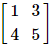does not exist.
Reason(R) :The matrix is non singular.

Solution:
QUESTION: 5

If the line 3x-4y=λ touches the circle x2+y2-4x-8y-5=0, λ  can have the values

Solution:
QUESTION: 6

The length of the tangent from (0,0) to the circle 2x2 + 2y2 + x - y + 5 = 0 is

Solution:
QUESTION: 7

The differential equation which represents the family of plane curves y=exp. (cx) is

Solution:

y = ecx
dy/dx = c. ecx
y' = cy

QUESTION: 8

If sin y = x sin (a + y), then (dy/dx) =

Solution:
QUESTION: 9

The fundamental period of the function f(x) = 2 cos 1/3(x - π) is

Solution:
QUESTION: 10
If A, B, C are represented by 3 + 4i, 5 - 2i, -1 + 16i respectively, then A, B, C are
Solution:
QUESTION: 11
Which of the following is not a statement ?
Solution:
QUESTION: 12
In the following question, a Statement-1 is given followed by a corresponding Statement-2 just below it. Read the statements carefully and mark the correct answer-
Consider the planes 3x – 6y – 2z = 15 and 2x + y – 2z = 5.
Statement-1:
The parametric equations of the line of intersection of the given planes are
x = 3 + 14t, y = 1 + 2t, z = 15t.
Statement-2:
The vector 14î+2ĵ+15k̂ is parallel to the line of intersection of given planes
Solution:
QUESTION: 13

In the following question, a Statement-1 is given followed by a corresponding Statement-2 just below it. Read the statements carefully and mark the correct answer-
Tangents are drawn from the point (17,7) to the circle x2+y2=169.
Statement-1:
The tangents are mutually perpendicular.
Statement-2:
The locus of the points from which mutually perpendicular tangents can be drawn to the given circle is x2+y2=338.

Solution: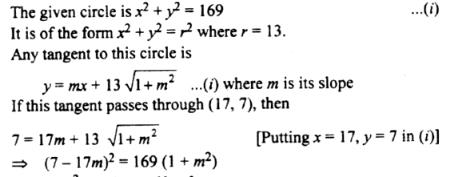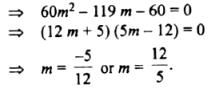Clearly, m1m2 = - 1.
Hence, the two tangents arc mutually perpendicular.
Statement 1 is true.
Now, the locus of the point of intersection of two mutually perpendicular tangents to the circle x2 + y2 = r2 is the director circle i.e. the circle x2 +y2 = 2r2
For the given circle r = 13. ..
Its director circle is x2 + y2 = 338.
Hence, statement 2 is true and  a cogect explanation of statement as the point (17, 7) lies on the director circle of the circle (i).

QUESTION: 14

The value of a for which the system of equations
a3x+(a+1)3y+(a+2)3z = 0
ax+(a+1)y+(a+2)z = 0
x+y+z = 0
has a non-zero solution, is

Solution:

The system of equation has a non-zero solution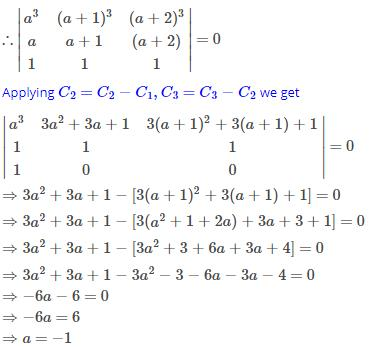QUESTION: 15

The pole of the line 2x + 3y − 4 = 0 with respect to the parabola y2 = 4 x is

Solution:
QUESTION: 16

If nC12=nC8, then n=

Solution:QUESTION: 17

The chance of getting a doublet with 2 dice is

Solution:

Total outcomes = 36
Doublet are 6 (1,1),(2,2),(3,3),(4,4),(5,5),(6,6)

Probability of getting doublet = 6/36
= 1/6

QUESTION: 18

If cov. (x, y) = 0, then ρ(x, y) equals

Solution:

Covariance is a quantitative measure of the extent to which the deviation of one variable from its mean matches the deviation of the other from its mean. It is a mathematical relationship that is defined as:

Cov(X,Y) = E[(X − E[X])(Y − E[Y])]

Correlation between two random variables, ρ(X,Y) is the covariance of the two variables normalized by the variance of each variable. This normalization cancels the units out and normalizes the measure so that it is always in the range [0, 1]:QUESTION: 19

The two opposite vertices of a square on xy-plane are A(-1,1) and B(5,3), the equation of other diagonal (not passing through A and B) is

Solution:

Given: Here,AB is the diagonal of square.
The vertices of a square A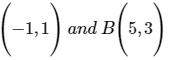Let the mid−point of AB be EThen coordinates of E are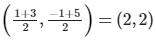Therefore equation of other diagonal is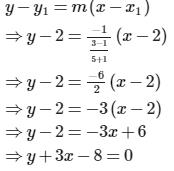QUESTION: 20

If the normal to the curve y=f(x) at the point (3,4) makes an angle 3π/4 with the positive x-axis, then f'(3)

Solution:

Given y = f(x)

differentiating w.r.t x

y' = f'(x) which is the slope of the tangent

Hence the slope of the normal is - 1/f'(x) = 3pi/4 = -1

therefore f'(x) = 1

Hence f'(3) = 1

*Answer can only contain numeric values
QUESTION: 21

Assume e-4/5 = 2/5. If x, y satisfy, y = ex and the minimum value of (x2 + y2) is expressed in the form of m/n then (2m - n)/5 equals (where m & n are coprime natural numbers)

Solution: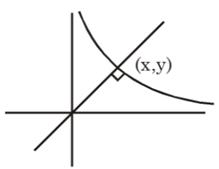OP2 = x2 + y2
y = ex, y' = ex,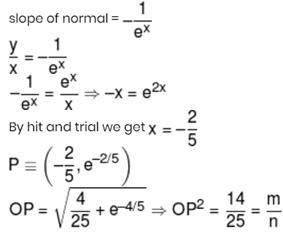*Answer can only contain numeric values
QUESTION: 22

Let ƒ(x) be non-constant thrice differentiable function defined on (–∞, ∞) such that ƒ(x) = ƒ(6 – x) and ƒ'(0) = 0 = ƒ'(2) = ƒ'(5). If 'n' is the minimum number of roots of (ƒ"(x))2 + ƒ'(x)ƒ"'(x) = 0 in the interval x ∈ [0, 6] then sum of digits of n equals

Solution:

ƒ(x) = ƒ(6 – x)
⇒ ƒ'(x) = –ƒ'(6 – x) .... (1)
put x = 0, 2, 5
ƒ'(0) = ƒ'(6) = ƒ'(2) = ƒ'(4) = ƒ'(5) = ƒ'(1) = 0
and from equation (1) we get ƒ'(3) = –ƒ'(3)
⇒ ƒ'(3) = 0
So ƒ'(x) = 0 has minimum 7 roots in
x ∈ [0, 6] ⇒ ƒ"(x) has min 6 roots in x ∈ [0,6]
h(x) = ƒ'(x).ƒ"(x)
h'(x) = (ƒ"(x))2 + ƒ'(x) ƒ"'(x)
h(x) = 0 has 13 roots in x ∈ [0, 6]
h'(x) = 0 has 12 roots in x ∈ [0, 6]

*Answer can only contain numeric values
QUESTION: 23

If a, b, c, x, y, z are non-zero real numbers and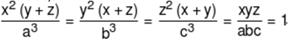then the value of (a3 + b3 + c3 + abc) equals

Solution:

x2(y + z)y2(z + x)z2(x + y) = a3b3c3 = x3y3z3
⇒ (x + y) (y + z) (z + x) = xyz
⇒ x2(y + z) + y2(z + y) + z2(x + y) + xyz = 0
⇒ a3 + b3 + c3 + abc = 0

*Answer can only contain numeric values
QUESTION: 24

If the co-ordinate of the vertex of the parabola whose parametric equation is x = t2 – t + 1 and y = t2 + t + 1, t ∈ R is (a, b) then (2a + 4b) equals

Solution:

x = t2 – t + 1    .... (1)
y = t2 + t + 1    .... (2)
y – x = 2t  &  x + y = 2(t2 + 1)
________on elminating 't' we get
⇒ (x + y – 2) = 2(y - x)/22
(x – y)2 = 2(x + y – 2)
Axis : x – y = 0
Tangent at vertex : x + y – 2 = 0
Vertex : (1, 1) = (x, y)

*Answer can only contain numeric values
QUESTION: 25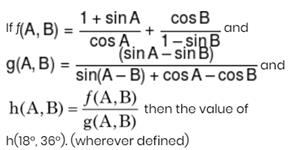Solution: